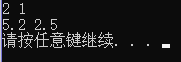# 初见C++函数模板

WARNING：函数的重载 !=函数模板

//函数模板的声明 关键字typename = class(旧版本)， T可以重复使用，生命周期是这个函数
//正常声明函数 void... 参数可以为为自定义的 参数类型 也可以是 其他的数据类型
template <typename T> void swap_show(T&, T&);

//函数的定义 将template <typename T>置于第一行说明是一个函数模板 也可以放在同一行
template <typename T>
void swap_show(T& a, T& b)
{
function body
}


#include <iostream>
using namespace std;

//函数模板声明
template <typename T> void swap_show(T&, T&);

int main()
{
int a = 1, b = 2;
swap_show(a,b);
double c = 2.5, d = 5.2;
swap_show(c,d);
system("pause");
return 0;
}

//函数模板的定义
template <typename T>
void swap_show(T& a, T& b)
{
T temp;
temp = a;
a = b;
b = temp;
cout << a  << ' '<< b << endl;
}11-19130
03-071万+03-02449
12-141153
09-143万+
08-0443
07-092411
05-2865
09-13198